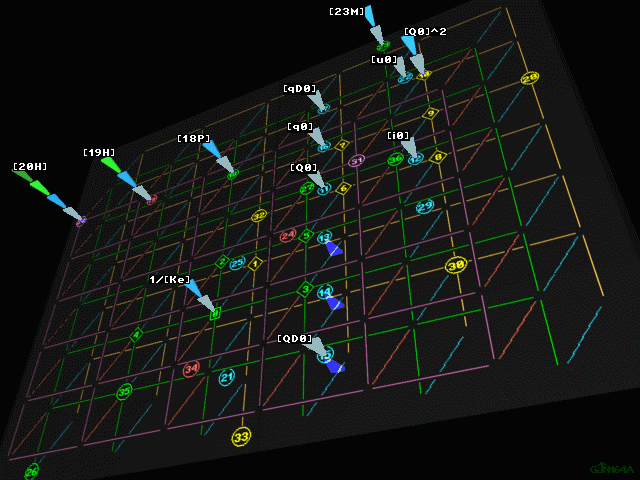```1280 X 960 GIF         Values table          Grayscale print

Tutorial back                           Symbol key, Tutorial nextColor print      1280 X 960 GIF      B & W print

[Ke] = 1/[Atomic unit of permittivity] = 1/{[4Pi][k]} , with units

[k] = electric constant, formerly called permittivity of the vacuum,

As an alternative view to GJN017 below, the picture immediately
above, GJN163, allows the portrayal of vectors into the fourth
dimension, as in the top graphic above.  To travel one wedge of
displacement toward the background (into or behind the plane where
the 3D vector space is depicted) is to multiply by a factor of
[Meters/Farad]^[1/2] , when speaking conceptually and only in terms
of units, or it is to multiply by the quantity [Ke]^[1/2] , when
speaking both numerically and in terms of units.  Represented by a
single wedge expanding toward the viewer, change of position toward
the foreground and out of the depiction plane represents division
by that factor.  Multiply in or divide out by [Ke] for each two
wedges of dispacement in the fourth dimension of the abstract space:

In the top graphic, start at {[Q0]^2} , the plot point in the 4D
space for [the atomic unit of charge]^2 , multiply by [Ke] , and
arrive at the plot point, back within the 2D depiction plane at
location *10* , the plot point for the product {[hB][v0]} with [hB]
as atomic unit of action and [v0] as atomic unit of velocity:

{[hB][v0]} = {[Q0]^2}[Ke] = {[Q0]^2}/{[4Pi][k]} ,

start at [QD0], the plot point extended into 4D space representing
the atomic unit of charge density, displace one wedge toward behind
the depiction plane, mutiplying by {[Ke]^.5} and arrive at location
*15* back within the plane:

{[Ke]^.5}[QD0] = {[Me]^.5}{[a0]^-1.5}[2af] ,

with [Me] as atomic unit of mass, [a0] as atomic unit of length, and
[2af] as the atomic unit of frequency.Color print      1280 X 960 GIF      B & W print

```